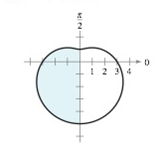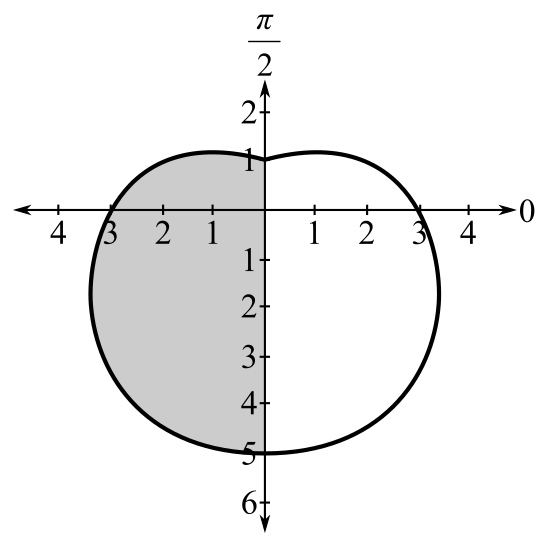Chapter 10.5, Problem 3E

Chapter
Section
Textbook Problem

# Area of a Polar Region In Exercises 3-6, write an integral that represents the area of the shaded region of the figure. Do not evaluate the integral. r = 3 − 2 sin θTo determine

To calculate: The required integral that represents area of shaded region:Explanation

Given:

The polar equation is r=32sinθ and graph of area of shaded region as shown below:

Formula used:

Area of shaded region of polar equations r=f(θ) between the radial lines θ=α and θ=β is

A=12αβ[r]2dθ

Calculation:

Consider the provided function r=32sinθ

From the given graph the area of shaded region lies between θ=π2 to θ=3π2

### Still sussing out bartleby?

Check out a sample textbook solution.

See a sample solution

#### The Solution to Your Study Problems

Bartleby provides explanations to thousands of textbook problems written by our experts, many with advanced degrees!

Get Started